# Using Generative Adversarial Networks to Create Dog Images

By: Zachary Arredondo, Kumaran Arulmani, Rahul Butani, Rishab Chander, Nathan Chin, Wenran Lu

GitLab Repository: https://gitlab.com/zarredondo/dogganit

## Introduction:

Our research focuses on generating square images of dogs using Deep Convolutional Generative Adversarial Networks (DCGANs). To improve the quality of the images generated, we tried a few techniques such as applying different loss functions, tuning hyper-parameters, and incorporating additional convolutional layers. Additionally, we scored our models using the Fréchet Inception Distance (FID) to analyze their performance. Our major goal was to improve the quality of the generated images and make them more realistic looking.

Motivation:

Inspired by the celebrity images generated using GANs in class, we decided to explore more intriguing applications for GANs. After searching through Kaggle for competitions and datasets, we found a competition called Generative Dog Images. As the competition was closed, we choose to use the dataset it involved (the Stanford Dogs Dataset) and develop our own scoring metrics for evaluating our models’ performance. All in all, the challenge was attractive to us as it seemed like a good way to develop a deeper understanding of the various GAN models and their uses. Generating dog pictures was definitely a nice bonus too.

Background:

GANs have become increasingly popular over the last few years. First introduced by Ian Goodfellow and other researchers at the University of Montreal in 2014, GANs have great potential thanks to their ability to mimic distributions of data. An analogy to help explain GANs is that of a counterfeit artist and an investigator. The counterfeiter repeatedly tries to forge art while the investigator tries to identify the counterfeits. The investigator is reprimanded when they allow forgeries to slip through and the counterfeiter is punished when their forgeries are caught by the investigator. Through this cycle both the investigator and counterfeiter gradually improve.

In an actual GAN, the counterfeit artist is represented by a generator network and the investigator by a discriminator network. The generator takes in a latent noise vector and attempts to generate an image (or sound, data, etc. — we say images because that’s what we used our GANs for but they can actually be used to model any kind of data), by attempting to model P(X|Y), where X represents the features, and Y represents a class. In other words, the generator attempts to model the distribution of an individual class. The generated image is then passed to the discriminator along with a stream of images from the ground-truth data set (non-generated images; i.e. real dogs). Ultimately, the discriminator models P(Y|X) and we expect the discriminator to accurately classify the ground-truth images as real and the generated images as fake. These two models exist in a double feedback loop. The discriminator is in a feedback loop with the ground-truth data set and the generated images, while the generator is in a feedback loop with the discriminator.

For this particular project, we decided to use a deep convolutional generative adversarial network (DCGAN), a variant of the typical GAN architecture described above. A DCGAN utilizes Deep Convolutional Layers (see Figure 4) in the generator, as opposed to fully-connected layers. Having convolutional layers allows for better spatial correlation, which is a reason why DCGANs are well-suited to images and videos.

Data:

Training a GAN requires a large amount of data. To avoid the pain and effort of self-curating a balanced dog image dataset, we decided to use the Stanford Dogs Dataset, a freely available corpus comprised of 20,580 annotated dog images across over 120 breeds. However, this well-classified dataset ended up being having some shortcomings. We discovered that there was little consistency of dog position and size across the images. Some images had a dog as the centerpiece of the image with the majority of the area being occupied by the dog while other images had their dog subjects off to the side or in the distance. This, combined with noisy backgrounds (i.e. massive color differences between images of the same dog breed), was challenging because it resulted in large intra-class variation and made it harder for the generator to hone in on the features we wanted to emphasize.

Data Processing:

In order to adjust the size of our images, we used the ImageFolder dataset class to load, transform, and eventually display images. As the snippet below illustrates, we first reshape input images to a size of 64 by 64 pixels and then convert the image to a normalized tensor.

The result below visualizes some of the training images. It is clear that some images contain entire dogs while some images have only portions of dogs due to cropping and reshaping. This is not optimal but was as best as we could given our limited resources.

Our Implementation:

We started by using the DCGAN architecture provided in the DCGAN PyTorch tutorial, which in turn, was based on Algorithm 1 from a paper by Ian Goodfellow. The generator consists of a series of strided 2D convolutional transposes, 2D batch normalizations, and ReLU activations. Figure 4 shows a generator network with 5 convolutional layers.

We largely wrote our models as Jupyter Notebooks with a roughly similar interface for training, scoring, and checkpointing to make it easy to train and compare our various models. Commonly used routines (such as scoring functions) were factored out into a module that the notebooks import and use. We also experimented with regular Python scripts for our models so that we could run them on headless server hardware (i.e. the ECE Linux hardware) but ultimately this was abandoned as the performance gains to be had from running on the servers available to us were negligible at best. The README of our repo has more information about the particulars of the project and the models that we ended up testing. The table from the README is also copied below:

The original architecture consists of 5 layers, including the first layer (denoted “Project and reshape” in Figure 4), which maps the latent vector to the first set of feature maps. Each feature map in the first set has height and width 4, and these dimensions double in each subsequent layer, ending with an output of height and width 64.

Our first attempt to tweak the original architecture was to introduce an additional convolutional layer. This is the model known as “DCGAN 2” in our repo and in the table above (Figure 5). We inserted a layer at the start that mapped the latent vector to feature maps of size 2 by 2, instead of 4 by 4. We also adjusted the following layer so that it mapped from feature maps of size 2 by 2 to feature maps of size 4 by 4. We give an illustration of the addition of this layer in Figure 5.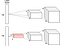Figure 5. Insertion of an additional set of feature maps, each of height 2 and width 2.

Adding this additional layer and set of feature maps was instructive in that it forced us to account for the relationship between input parameters like kernel_size, padding, and stride and the input and output dimensions. The PyTorch documentation for ConvTranspose2d provides a formula to calculate output height based on input height and the other parameters (the calculation is identical for width in our case):

Figure 7 shows the code snippet from the original model and the corresponding calculation that describes the mapping from the latent vector (whose height is 1) to the first feature map (whose height is 4):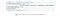Figure 7. Code snippet showing the original ConvTranspose2d call mapping the latent vector to the 4 by 4 feature map

We adjusted kernel_size from 4 to 2 in order to yield a feature map of height 2. Figure 8 shows the modified code snippet and the updated calculation.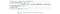Figure 8. Code snippet showing the modified ConvTranspose2d call, which maps the latent vector to a 2 by 2 feature map.

In the next layer, we restored kernel_size to 4 but changed stride and padding to 2 and 1, respectively. Figure 9 shows the code snippet and corresponding calculation for the layer between the 2 by 2 and 4 by 4 feature maps.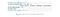Figure 9. Code snippet showing new ConvTranspose2d call, which maps 2 by 2 feature maps to 4 by 4 feature maps.

After inserting an additional layer of size 2 by 2 to both the generator and the discriminator, we started training our model with a Binary Cross Entropy loss (BCELoss) function. This corresponds to the model referred to as “BCE Logits” in our repo and the table above (Figure A). The performance of generator loss and discriminator loss using a BCELoss function is shown in the figure below.

The results of using an additional layer in both the generator and discriminator models are shown in the figure below. As we can observe from the figure, the images generated through the DCGAN models have some basic dog characteristics such as noses, mouths, tails and some color patterns. However, compared to the real images, these images have blurred edges and irregular color blocks.

Tuning parameters

After testing models with an additional layer on the generator and discriminator networks, we then tried to tune certain input parameters and activation functions to improve the quality of our results. By default, we use the following values for the following tunables (as also shown in the image below):

1. 64 by 64 for the image size
2. 128 for the batch size
3. 100 for the z latent vector
4. 64 for the size of feature maps in the generator
5. 64 for the size of feature maps in the discriminator
6. 0.0002 for the learning rate for the optimizers
7. 0.5 for the beta hyperparameter for the Adam optimizers

This default model (using the parameter values shown above) corresponds to the model known as “DCGAN 1” in our repo and in the table above (Figure A).

Our first approach was to change the batch size, the size of training images selected, and train the model over epochs. This maps to the model called “Leaky” in our repo and in the table above (Figure A). We tried smaller batch sizes for two main reasons. First, smaller batch sizes give a regularizing effect with lower generalization error. Second, smaller batch sizes help to reduce the training time in our case. It is true that large batch sizes allow computational speedups from the parallelism of GPUs. However, our project only uses 1 GPU and thus small batch sizes such as 32 work well for our model.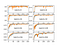Figure 13. The performance with changing batch size (from the internet)

Additionally, in this model we choose to use LeakyReLU on every layer of the generator’s network (hence, “Leaky”). When using regular ReLU activation functions, a potential problem is that a ReLU neuron caps values in the negative range to 0 causing it to thus always output a gradient of 0 in such cases. It’s unlikely for the neuron to recover in this situation and hence it becomes useless since it does not play any role in discriminating the input. Over time, we end up with a large part of our network doing nothing. To combat this, we decided to use LeakyReLU. Because LeakyReLU has a very slight positive slope (as shown below in Figure 14) for negative inputs, it doesn’t have this same failure mode that causes parts of the graph to go effectively unused.

Using different loss functions

The choice of loss function may also have a dramatic impact on training a model. Our initial implementation (“DCGAN 1”) uses a BCELoss function to train the generator and the discriminator. We attempted to use alternative loss functions to make a comparison between those results as well as to find the best approach to improve our model performance.

BCELoss

The default BCELoss function we used measures the binary cross-entropy between the target and the output. The figure below (Figure 15) shows the loss during training when the BCELoss function was used.

The BCELoss function was used in the DCGAN 1, 2, 3, and 4 models as well as the Leaky and Correct models (see the table above — Figure A).

BCEWithLogitsLoss

Unlike the default BCELoss function which receives the output of a sigmoid layer as its input, the BCEWithLogitsLoss function instead takes logits as its input and applies a sigmoid layer internally. After training for a few epochs using BCEWithLogitsLoss function, we obtained a discriminator loss of 1.0064 and a generator loss of 0.6931. The overall performance using this loss function is shown in Figure 16. Clearly, this approach failed to generate improved results.

KL Divergence Loss

KL Divergence Loss is used to measure distance for continuous distributions and is also used when performing direct regression over discretely sampled continuous output distributions. It expects the target y to have the same size as the input x. In our model named “KLDivLoss”, we were unable to obtain produce meaningful loss values, as seen in the figure below.

Smooth L1 Loss

The Smooth L1 loss function, also known as The Huber Loss, is a combination of the L1 and L2 Loss functions. While the L1 loss function measures the mean absolute error (MAE) between input x and target y, the L2 loss function measures the Mean Squared Error (MSE). The Smooth L1 loss function uses MSE if the absolute error between element xi and yi falls below 1 and MAE otherwise. Ideally, the advantage of using Smooth L1 loss function is that it produces steady gradients when x has large values and less fluctuation when x has small values.

Our model, named “SmoothL1”, illustrates unintended results in the figure below.

Measuring Performance Metrics:

Though a fair number of our models can be reasonably evaluated by qualitative means (i.e. just by looking at the produced images, some GAN models are obviously better than others), we still wanted a quantitative method of scoring the models.

In searching for such a method we came across the Inception Score (IS) and the Fréchet Inception Distance (FID): two methods of evaluating the images produced by GANs.

Both of these involve using an InceptionV3 model (a popular performant image classification model), though in different ways, as shown below (Figure 18).

Inception Score actually uses the InceptionV3 model in its entirety: the generated images are fed through an InceptionV3 model and the outputs (classes with probabilities) are then used to compute the conditional and marginal probabilities of each image (marginal probabilities are found by averaging the conditional probabilities of the images in a group). The Inception Score is meant to measure quality and diversity; quality being how realistic individual images look (i.e. does it look like a dog?) and quantity being how well the spread of generated images cover the spread of actual objects (i.e. do we make images for all the different dog breeds? did we succumb to mode collapse?). For this score, the conditional probability is a stand in for quality and marginal for diversity. Finally, the KL Divergence (this varies depending on the flavor of Inception Score) is calculated for the conditional and marginal probabilities across all of the images in the generated batch.

This works well and has been shown to perform similarly to human based classification of the generated instances (in one research paper, an Amazon Mechanical Turk team’s feedback was compared to IS scores and found to be roughly similar). However, one downside to IS is that actual statistics regarding the images and their features are ignored. For example, the IS does not encode or enforce characteristics such as, for example, images with dogs typically having four legs. The Fréchet Inception Distance (FID) aims to combat this.

Unlike IS, the Fréchet Inception Distance does not use the entire InceptionV3 model; instead it stops before classification begins at one of the global spatial pooling layers (typically the last one, yielding a vector of 2048 elements). The output from the model is then a computer vision specific feature vector which encodes spatially aware properties of the image. In calculating the Fréchet Inception Distance, this vector is calculated for a batch of real images and generated images. The distribution of vectors for each of the real and generated image sets are flattened into parameters representing the respective distribution (mean and covariance). These parameters are then used to calculate the Fréchet distance between the two vectors and also the Fréchet Inception Distance.

As mentioned, because of its use of the spatially aware feature vector from the InceptionV3 model, FID is able to do a better job ensuring inherent properties of the images being generated are present in the output. As such, we choose to score our models with FID.

Below is a table (from the README as well) of the FID scores (lower is better) of the models that successfully completed multiple epochs and did not involve altering the output image size (due to a limitation in the transformation step, our FID scoring routines cannot currently properly handle images that aren’t 64 by 64 pixels in size).

These results roughly corroborate our qualitative assessments of the performance of our models.

Additionally, below are some animated progressions of our models as they were being trained. Careful: some of the early dogs are quite grotesque.